# Boyles Law If n and T are constant

• Slides: 6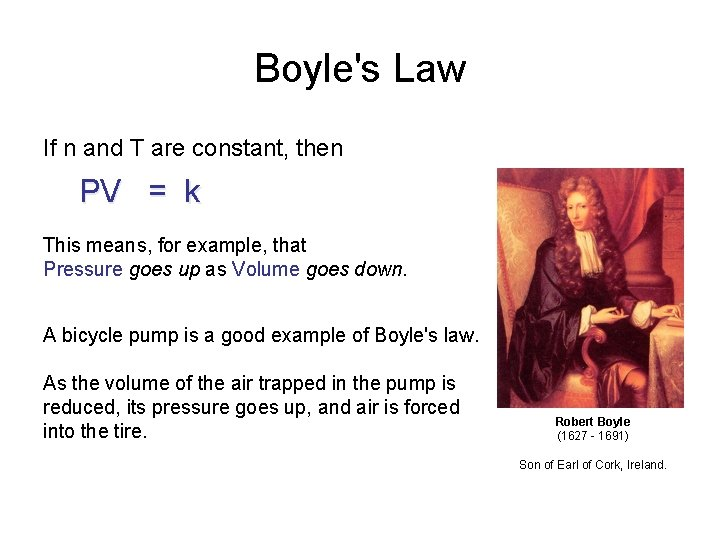Boyle's Law If n and T are constant, then PV = k This means, for example, that Pressure goes up as Volume goes down. A bicycle pump is a good example of Boyle's law. As the volume of the air trapped in the pump is reduced, its pressure goes up, and air is forced into the tire. Robert Boyle (1627 - 1691) Son of Earl of Cork, Ireland.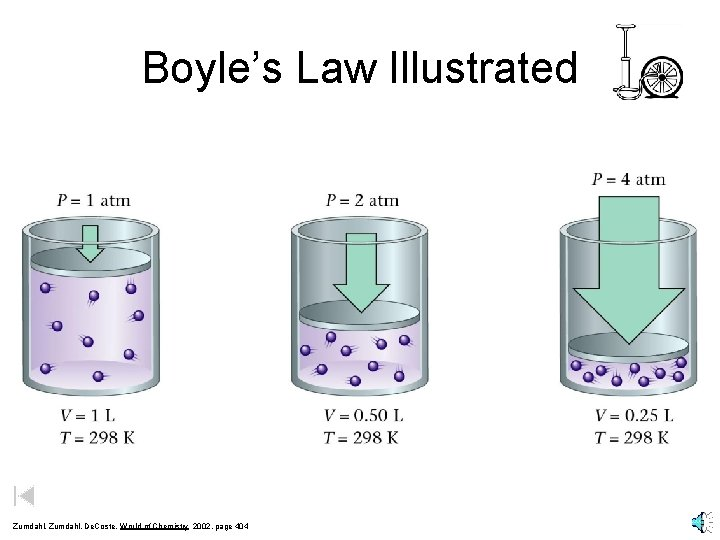Boyle’s Law Illustrated Zumdahl, De. Coste, World of Chemistry 2002, page 404Pressure vs. Volume for a Fixed Amount of Gas (Constant Temperature) 600 Volume (m. L) 500 400 300 200 100 200 300 Pressure (KPa) 400 500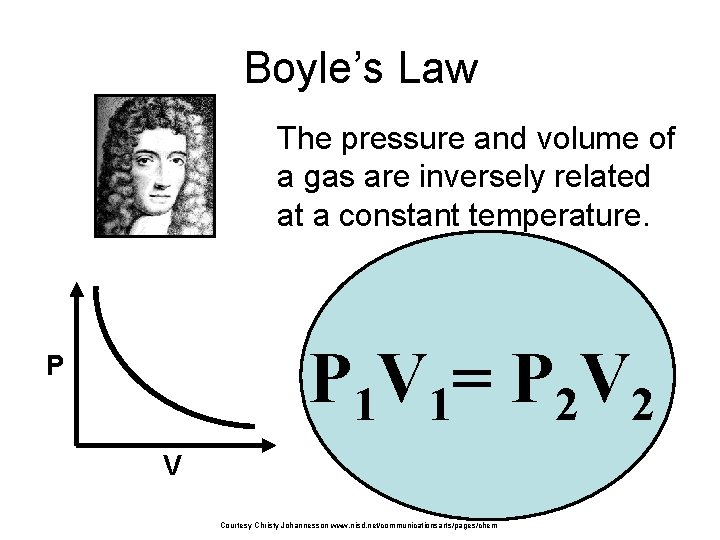Boyle’s Law The pressure and volume of a gas are inversely related at a constant temperature. P 1 V 1= P 2 V 2 P V Courtesy Christy Johannesson www. nisd. net/communicationsarts/pages/chem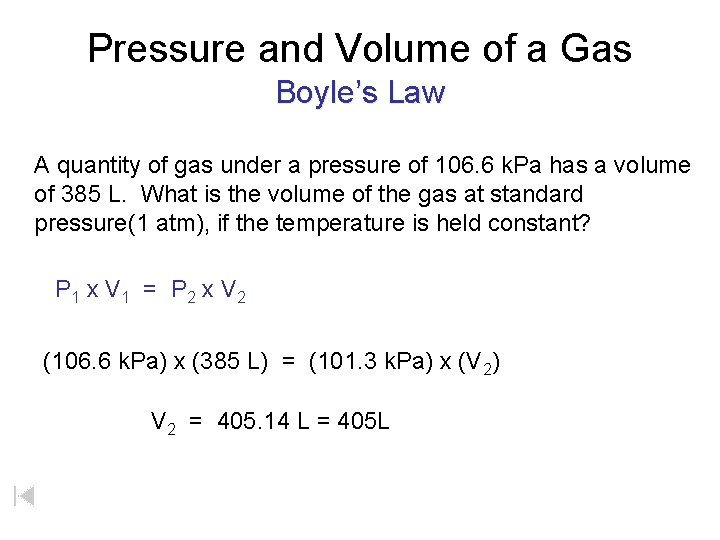Pressure and Volume of a Gas Boyle’s Law A quantity of gas under a pressure of 106. 6 k. Pa has a volume of 385 L. What is the volume of the gas at standard pressure(1 atm), if the temperature is held constant? P 1 x V 1 = P 2 x V 2 (106. 6 k. Pa) x (385 L) = (101. 3 k. Pa) x (V 2) V 2 = 405. 14 L = 405 L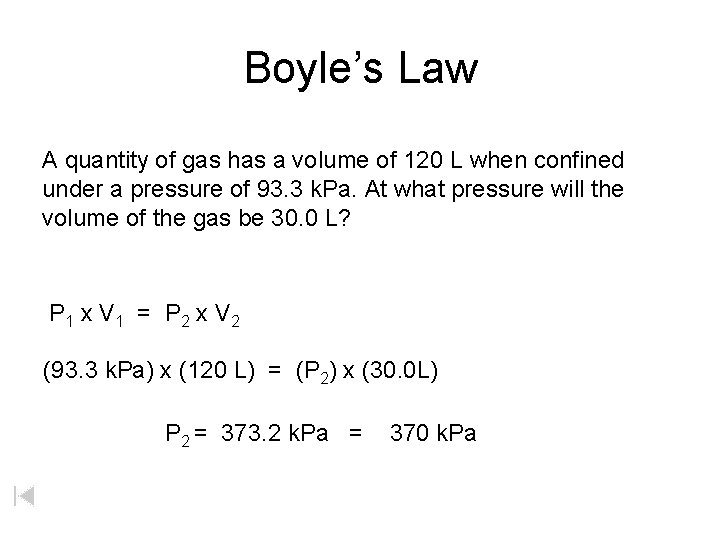Boyle’s Law A quantity of gas has a volume of 120 L when confined under a pressure of 93. 3 k. Pa. At what pressure will the volume of the gas be 30. 0 L? P 1 x V 1 = P 2 x V 2 (93. 3 k. Pa) x (120 L) = (P 2) x (30. 0 L) P 2 = 373. 2 k. Pa = 370 k. Pa